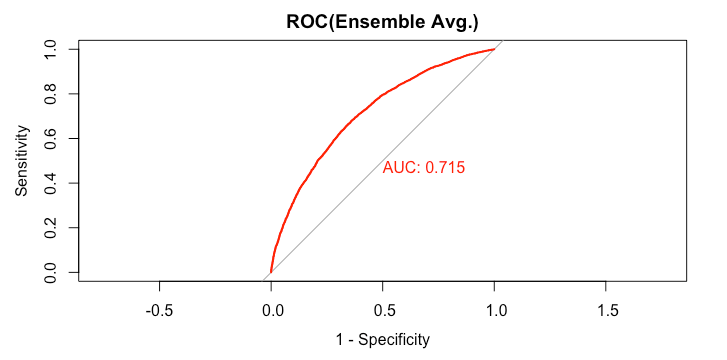# Predictive Modelling: Averaging Results from Multiple Models

Our final model is to combine the result of previous machine learning models and provide a single prediction by averaging probabilities from all previous models.

predict_loan_status_ensemble = predict_loan_status_logit +
predict_loan_status_svm +
predict_loan_status_rf +
predict_loan_status_xgb
predict_loan_status_ensemble = predict_loan_status_ensemble / 4
rocCurve_ensemble = roc(response = data_test$loan_status, predictor = predict_loan_status_ensemble) auc_curve = auc(rocCurve_ensemble) plot(rocCurve_ensemble,legacy.axes = TRUE,print.auc = TRUE,col="red",main="ROC(Ensemble Avg.)")> rocCurve_ensemble Call: roc.default(response = data_test$loan_status, predictor = predict_loan_status_ensemble)

Data: predict_loan_status_ensemble in 5358 controls (data_test$loan_status Default) < 12602 cases (data_test$loan_status Fully.Paid).
Area under the curve: 0.7147
>

predict_loan_status_label = ifelse(predict_loan_status_ensemble<0.5,"Default","Fully.Paid")
c = confusionMatrix(predict_loan_status_label,data_test$loan_status,positive="Fully.Paid") table_perf[5,] = c("Ensemble", round(auc_curve,3), as.numeric(round(c$overall["Accuracy"],3)),
as.numeric(round(c$byClass["Sensitivity"],3)), as.numeric(round(c$byClass["Specificity"],3)),
as.numeric(round(c\$overall["Kappa"],3))
)


We get the following performance:

> tail(table_perf,1)
model   auc accuracy sensitivity specificity kappa
5 Ensemble 0.715     0.65       0.637        0.68 0.275
>